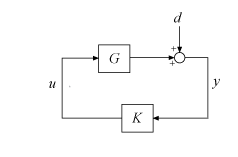# augw

Plant augmentation for weighted mixed-sensitivity H and H2 loop-shaping design

## Syntax

``P = augw(G,W1,W2,W3)``

## Description

example

````P = augw(G,W1,W2,W3)` computes a state-space model of an augmented LTI plant P(s) with the weighting functions W1(s), W2(s), and W3(s) penalizing the error signal, control signal, and output signal, respectively. `P` is the augmented plant of the following diagram.This control structure is used in mixed H∞ synthesis, which lets you design an H∞ controller by simultaneously shaping the frequency responses for tracking and disturbance rejection, noise reduction and robustness, and controller effort. For more information, see Mixed-Sensitivity Loop Shaping.```

## Examples

collapse all

Suppose you want to synthesize a stabilizing robust controller for the system of the following diagram. The controller must also reject disturbances injected at the plant output.The plant, `G`, is an unstable first-order system.

`G = tf(1,[1 -1]);`

To set up this problem for `hinfsyn`, insert a weighting function `W1` that captures the disturbance rejection goal, and another weighting function `W3` to enforce robustness. Specify these weighting functions as the inverses of the desired loop shapes for the sensitivity `S` and complementary sensitivity `T`, respectively. (See Mixed-Sensitivity Loop Shaping.)

For this example, choose `W1` with:

• Low-frequency gain of 100 (40 dB)

• 0 dB crossover at 0.5 rad/s

• High-frequency gain of 0.25 (`–`12 dB)

Choose `W3` to have the opposite low-frequency and high-frequency gains.

```W1 = makeweight(100,[1 0.5],0.25); W3 = makeweight(0.25,[1 0.5],100); bodemag(W1,W3)```For this example, do not specify a `W2` (no restriction on control effort). Construct the augmented plant, `P`.

`P = augw(G,W1,[],W3);`

G has one input and one output. The augmented plant has an additional input for the control signal, and additional outputs for each of the weights.

`size(P)`
```State-space model with 3 outputs, 2 inputs, and 3 states. ```

The inputs and outputs of `P` are grouped to keep track of the disturbance and control inputs and the error and measurement outputs. For example, example the output groups. Group `Y1` contains the two error outputs z, and group `Y2` contains the single measurement output.

`P.OutputGroup`
```ans = struct with fields: Y1: [1 2] Y2: 3 ```

You can now use `P` for control design. For example, use `hinfsyn` to design an ${\mathit{H}}_{\infty }$ optimal controller that meets the design requirements specified by `W1` and `W3`.

```[K,CL,gamma] = hinfsyn(P); gamma```
```gamma = 0.9946 ```

## Input Arguments

collapse all

Plant, specified as a dynamic system model such as a state-space (`ss`) model. `G` can be any LTI model. If `G` is a generalized state-space model with uncertain or tunable control design blocks, then `mixsyn` uses the nominal or current value of those elements.

Weighting functions, specified as dynamic system models. Choose the weighting functions `W1,W2,W3` to shape the frequency responses for tracking and disturbance rejection, controller effort, and noise reduction and robustness. Typically:

• For good reference-tracking and disturbance-rejection performance, choose `W1` large inside the control bandwidth to obtain small S.

• For robustness and noise attenuation, choose `W3` large outside the control bandwidth to obtain small T.

• To limit control effort in a particular frequency band, increase the magnitude of W2 in this frequency band to obtain small KS.

If one of the weights is not needed, set it to `[]`. For instance, if you do not want to restrict control effort, use `W2 = []`.

Use `makeweight` to create weighting functions with the desired gain profiles. For details about choosing weighting functions, see Mixed-Sensitivity Loop Shaping.

If `G` has NU inputs and NY outputs, then `W1,W2,W3` must be either SISO or square systems of size NY, NU, and NY, respectively.

Because S + T = I, `mixsyn` cannot make both S and T small (less than 0 dB) in the same frequency range. Therefore, when you specify weights for loop shaping, there must be a frequency band in which both `W1` and `W3` are below 0 dB.

## Output Arguments

collapse all

Augmented plant, returned as a state-space (`ss`) model. `P` can be any LTI model with inputs [w;u] and outputs [z;y]. `augw` groups the inputs and outputs of `P` using the `ss` properties `InputGroup` and `OutputGroup` such that:

• `P.InputGroup` has field `U1` containing the inputs corresponding to w, and field `U2` containing the inputs corresponding to u.

• `P.OutputGroup` has field `Y1` containing the outputs corresponding to z, and group `Y2` containing the outputs corresponding to e.

Here, {w;u} and {z;e} are the inputs and outputs of `P` in the following control system.## Tips

• For H or H2 synthesis, the models `G` and `W1,W2,W3` must be proper. In other words, they must be bounded as $s\to \infty$ (for continuous-time transfer functions) or $z\to \infty$ (for discrete-time transfer functions). Additionally, `W1,W2,W3` must be stable. The plant `G` must be stabilizable and detectable. Otherwise, the resulting `P` is not stabilizable by any controller.

## Algorithms

`augw` produces the augmented plant P(s) given by:

`$P\left(s\right)=\left[\begin{array}{cc}{W}_{1}& -{W}_{1}G\\ 0& {W}_{2}\\ 0& {W}_{3}G\\ I& -G\end{array}\right]$`

The partitioning is embedded using `P = mktito(P,NY,NU)`, which sets the `P.InputGroup` and `P.OutputGroup` properties as follows.

```[r,c] = size(P); P.InputGroup = struct('U1',1:c-NU,'U2',c-NU+1:c); P.OutputGroup = struct('Y1',1:r-NY,'Y2',r-NY+1:r); ```